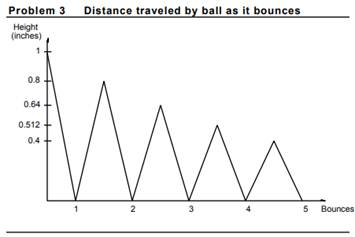## develop a program to compute and display some data on the bounces a ball will make when dropped from a given height.

A Bouncing Ball

While waiting for your date to show up, you idly bounced a tennis ball on the sidewalk. This gave you the idea to develop a program to compute and display some data on the bounces a ball will make when dropped from a given height. Forgetting your late date, you went home to solve this interesting problem. To simplify the problem, you assume the ball bounces in place; that is, it remains bouncing on the same spot and does not have any forward motion. The program will prompt the user for the initial height of the ball, the number of bounces to consider, and the ball’s elasticity (which must lie between 0 and 1). It will compute the height of each bounce based on the initial height and the elasticity of the ball, and display it. The program will also compute the total distance traveled, which is the sum of the up and down bounces, for the given number of bounces, and display it. The program will repeat this process until the user tells it to stop. If the ball is at height h, when it bounces, it reaches new height h’, which is computed by the formula h’ = h ´ resilience where resilience is expressed as the elasticity coefficient raised to the nth power, if n is the bounce number: resilience = elasticityn If the original height is H, then the first bounce height will be h 1 = H ´ elasticity With an elasticity in the range between 0 and 1, each bounce will be smaller than the preceding one, but never become 0. We will therefore stop the program after a specified number of bounces. The ball will travel the distance shown in Figure 10.11 (where the elasticity is 0.8) which we have drawn to help you. We have represented a forward motion for the sake of clarity (the ball bounces on the same spot).Each time the ball bounces, it travels twice the height of the bounce, so the total distance traveled is

H + 2h1 + 2h2 + 2h3 + 2h4 + …

The user will be prompted for the initial height in this way:

Give the height in inches from which the ball is dropped:

Then the user will be asked the number of bounces:

Give the number of times the ball will bounce:

And finally the user will be asked the elasticity of the ball:

Give the elasticity of the ball (between 0 and 1):

For each bounce the program will display the following:

On bounce 9 the ball rises to a height of 99.9 inches.

After the last bounce the program will display the following:

The total distance traveled by a ball with an elasticity of 0.999 when dropped from a height of 99.9 inches and after bouncing 9 times is 999 inches.

The user will be asked if the program is to continue: Another try?

### Determine the total amount of the loan based on the following parameters.

Python Input Statements   You are considering buying a condominium in the Tidewater Condominium Complex and have decided to estimate the total costs involved in applying for a loan. Since….

### Determine the total amount of the loan based on the following parameters.

Python Input Statements       You are considering buying a condominium in the Tidewater Condominium Complex and have decided to estimate the total costs involved in applying for a….

### Write a driver program to test your method scienceWriteln.

Define a utility class for displaying values of type double. Call the class DoubleOut. Include all the methods in the class DollarFormat, all the methods from the class OutputFormat ,….